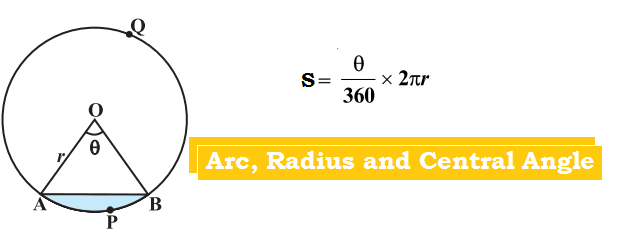# Relation between Arc, Radius and Central AngleAs shown in the diagram,

Consider a circle with centre O and AOB being the sector with sector angle θ and arc APB.

Length of the arc of a sector of angle θ, S

Solved Examples

1.If the angular diameter of the moon be 30′, how far from the eye a coin of diameter 2.2 cm be kept to hide the moon?

θ = 30′ =0.50

S= 2.2 cm

r= (S*360)/(2π*θ)

r= (2.2*360)/(2*3.14*0.5)cm= 252 cm